# Analysis of a cycle and its thermal performance

## Second law of thermodynamics

No real or ideal engine can turn all the heat introduced into mechanical work.

Therefore, only a fraction of the heat supplied by the combustion will be converted into work; This fraction represents the thermal performance of the engine. Then we define,

Ideal thermal performance.

Relation between the amount of heat transformed into useful work and the amount of heat supplied to the fluid.

As useful work equals the difference between the supplied heat Q1 and the subtracted heat Q2 we have:

Anyone can get a more complete idea of the thermal performance concept and gain familiarity with the interpretation of the diagrams by examining the sequence of transformations in P-V and T-S coordinates for an ideal cycle.

### Compression

The yellow zone (L2) represents the work done by the piston. The piston moves from the bottom dead center to the top dead center.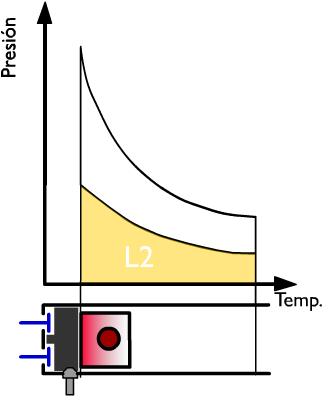### Combustion

The heat produced by the combustion is introduced instantaneously, since the cycle is at constant volume. The blue zone QI represents the heat supplied.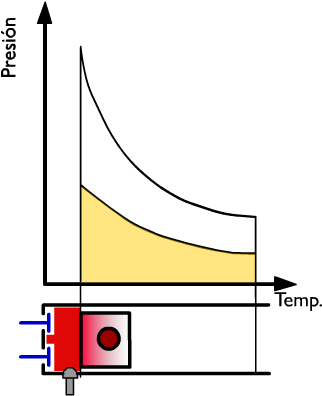-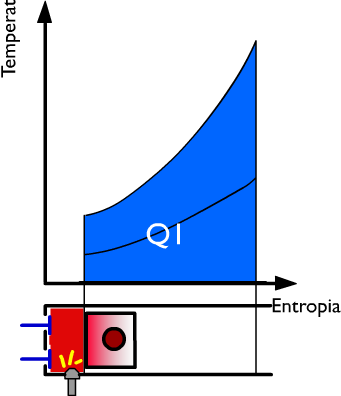### Expansion

The piston moves, by default, from the fluid pressure, from the top dead center to the bottom dead center. The LI work produced by the active fluid during expansion is represented in P-V coordinates by the dashed area between the upper adiabatic expansion line and the abscissa axis.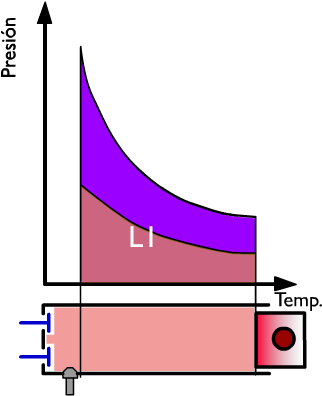-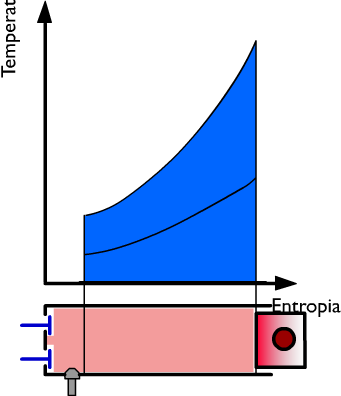### Escape

At this instant, the exhaust at constant volume follows and during this phase of the cycle the heat Q2 is subtracted while the pressure drops. On the right side, the heat Q is represented, in coordinates T-S, by the dark blue surface. In violet is represented the useful work and in light blue the used heat.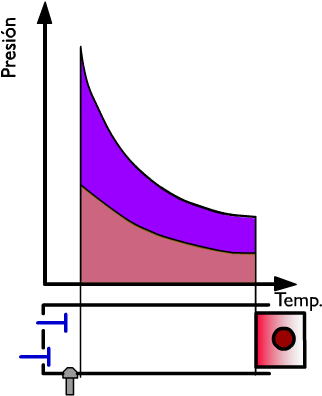-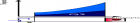As useful work, obviously, equal to the heat used, we can write: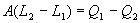In this way we can simplify the computation of the useful work and of the thermal yield of cycle using the values Q1 and Q2 instead of L1 and L2.

valoración: 2.3 - votos 17

Last review: February 1, 2016